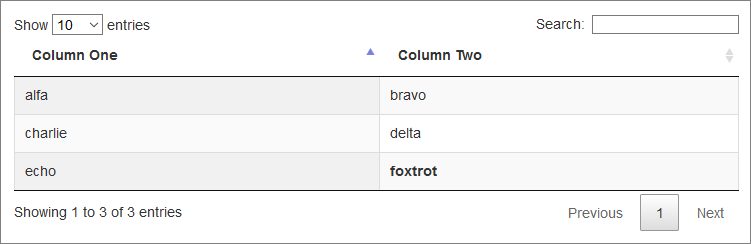See full details in this blog page.

A summary:

rows().every(), columns().every() and cells().every() - Use when you want to perform an API method on each selected item, but there is a performance penalty.

each() - similar to every() but doesn’t create a new API instance and doesn’t change the callback function’s scope. Lower level.

iterator() - For all other data types that the DataTables API can carry we use the each() to access the data in the instance.

The Table

We assume a simple table containing the following:It is defined as follows:

 1 2 3 4 5 6 7 8 9 10
Column OneColumn Two
alfabravo
charliedelta
echofoxtrot

The DataTables object is created as follows:

 1 2 3 4 5 6 var table = \$('#demo').DataTable({ "columns": [ null, null ] });

We then have the following various ways of accessing data in this table:

All Cell Data

Iterate all cell data as a JavaScript array of arrays:

 1 2 3 4 5 6 7 var allData = table.data(); for (var i = 0; i < allData.length; i++) { var rowData = allData[i]; for (var j = 0; j < rowData.length; j++) { console.log("row " + (i+1) + " col " + (j+1) + ": " + rowData[j]); } }

Output:

row 1 col 1: alfa
row 1 col 2: bravo
row 2 col 1: charlie
row 2 col 2: delta
row 3 col 1: echo
row 3 col 2: <b>foxtrot</b>

One Cell

Get only one cell - row 3 column 2:

 1 2 var oneSelectedCell = table.cell(2, 1); console.log(oneSelectedCell.data());

Output:

<b>foxtrot</b>

One Node

Get one cell’s <td> node - row 3 column 2:

 1 2 var oneSelectedCell = table.cell(2, 1); console.log(oneSelectedCell.node());

Note that this returns a native DOM element, not a jQuery object.

Using CSS

Get some cells using a css class name:

 1 2 3 4 var someSelectedCells = table.cells(".foo").data(); for (var i = 0; i < someSelectedCells.length; i++) { console.log(someSelectedCells[i]); }

Output:

bravo
charlie

The every() Function

Get all cells using the ‘every()’ function.

Two variations:

 1 2 3 table.cells().every( function () { console.log(this.data()); } );
 1 2 3 table.cells().every( function () { console.log(this.node().textContent); } );

Output:

row 1 col 1: alfa
row 1 col 2: bravo
row 2 col 1: charlie
row 2 col 2: delta
row 3 col 1: echo
row 3 col 2: <b>foxtrot</b>

Every Row

Similar to the above, but returns an array of data for each row:

 1 2 3 table.rows().every( function () { console.log(this.data()); } );

Output:

[ "alfa", "bravo" ]
[ "charlie", "delta" ]
[ "echo", "<b>foxtrot</b>" ]

Cell Data - No HTML

Get only one cell without the HTML tags - row 3 column 2:

 1 2 3 var oneSelectedCell = table.cell(2, 1); var node = oneSelectedCell.node(); console.log(node.textContent);

Output:

foxtrot

One Column

Get column 2’s data:

 1 2 3 4 var oneSelectedColumn = table.column(1).data(); for (var i = 0; i < oneSelectedColumn.length; i++) { console.log(oneSelectedColumn[i]); }

Output:

bravo
delta
<b>foxtrot</b>

Get column 2’s data via the nodes:

 1 2 3 4 var oneSelectedColumn = table.column(1).nodes(); for (var i = 0; i < oneSelectedColumn.length; i++) { console.log(oneSelectedColumn[i].textContent); }

Output:

bravo
delta
foxtrot

Row Iterator

Use a ‘row’ iterator to get row 2:

 1 2 3 table.rows(1).iterator( 'row', function ( context, index ) { console.log( \$( this.row( index ).node() ) ); } );

Column Iterator

Use a ‘column’ iterator to get each column:

 1 2 3 4 5 table.columns().iterator( 'column', function ( context, index ) { console.log( context ); console.log( index ); console.log( \$( this.column( index ) ) ); } );

The slice() Function

 1 2 3 4 5 6 7 8 9 table.rows().every( function ( ) { var data = this.data(); // get a subset of the row data, excluding the first cell // if the values are stored as strings, map then to numbers var values = data.slice(1).map(Number); // the ... operator expands the array into values for the // max() function to operate on: console.log( data + ': ' + Math.max( ...values ) ); } );